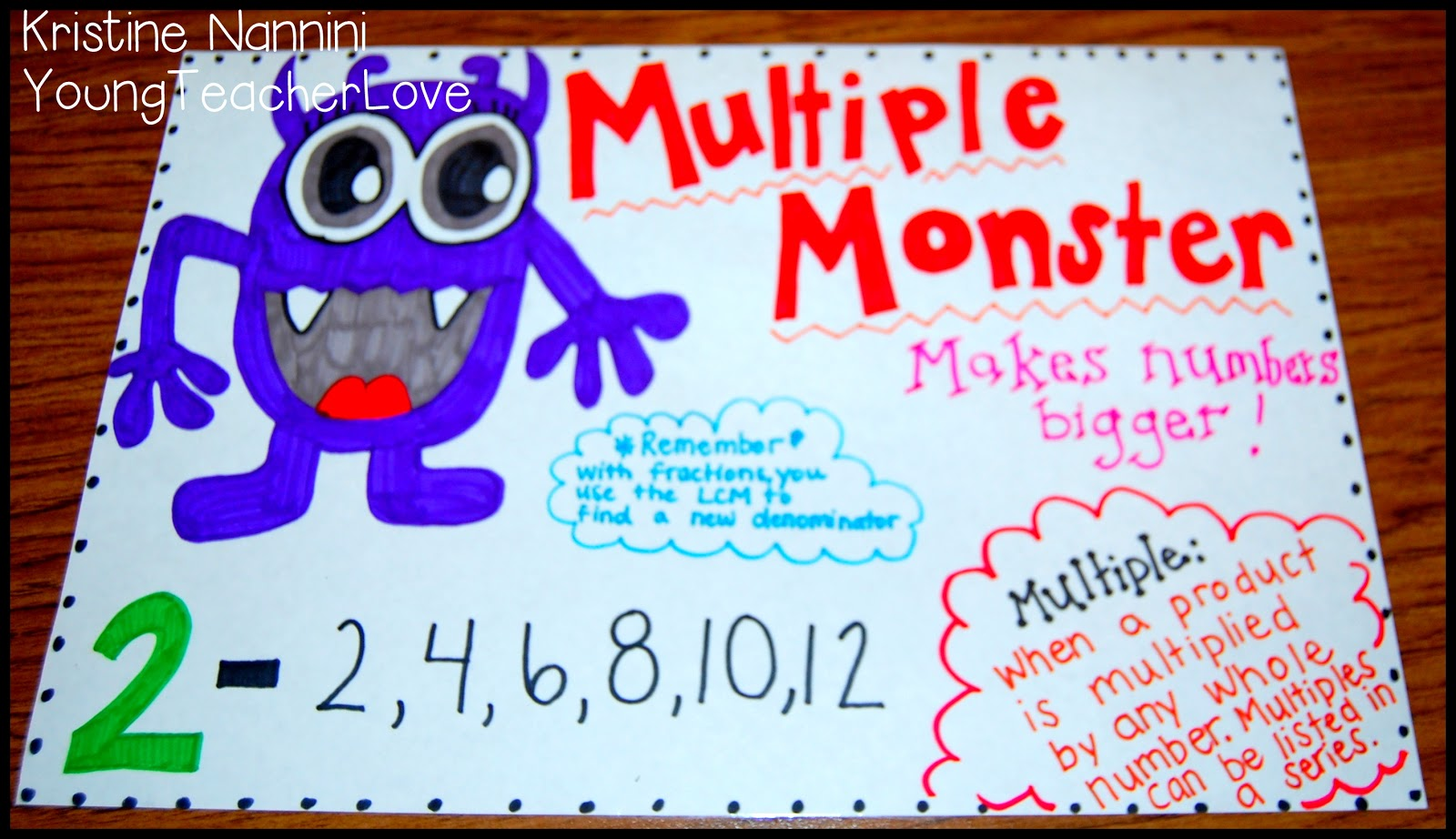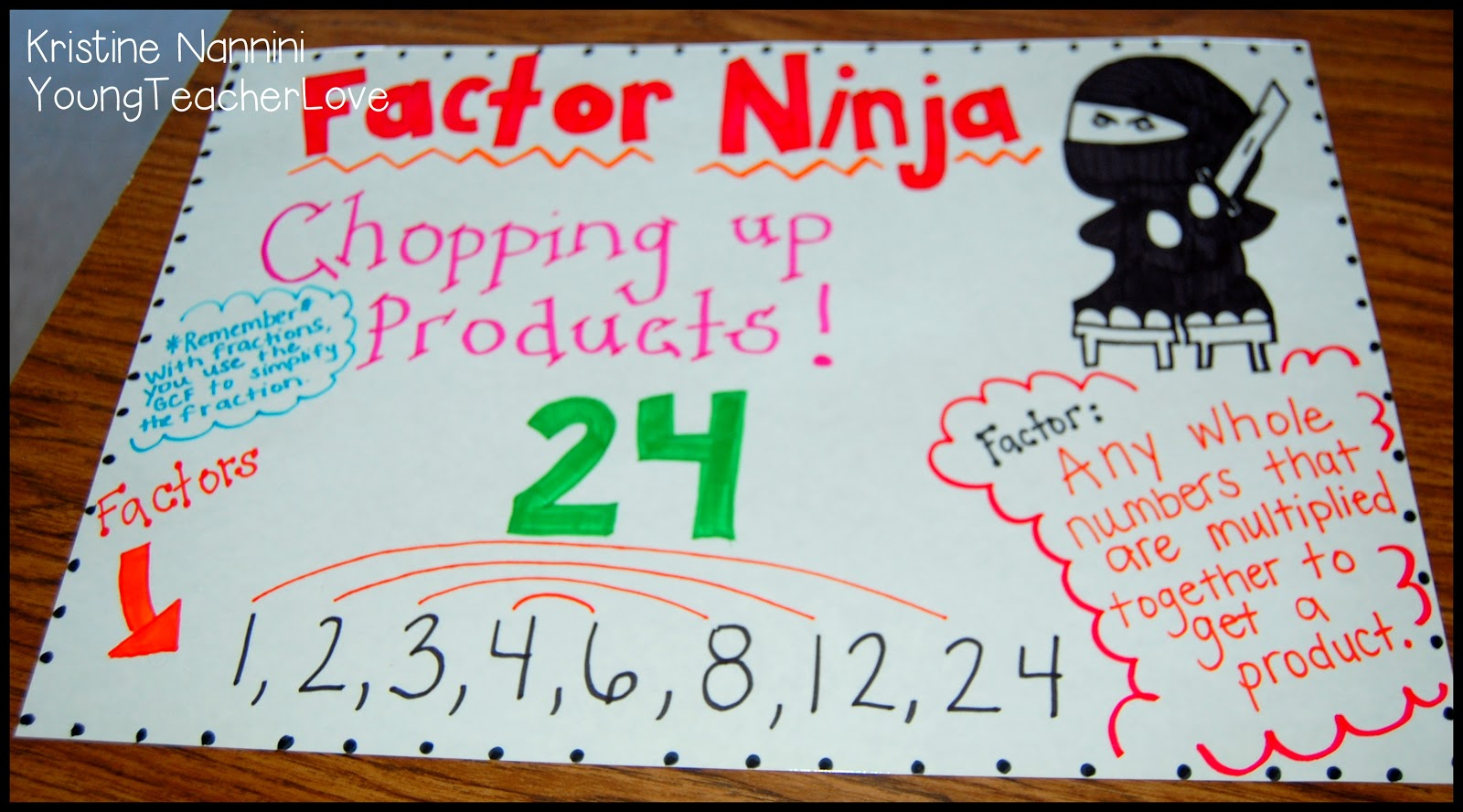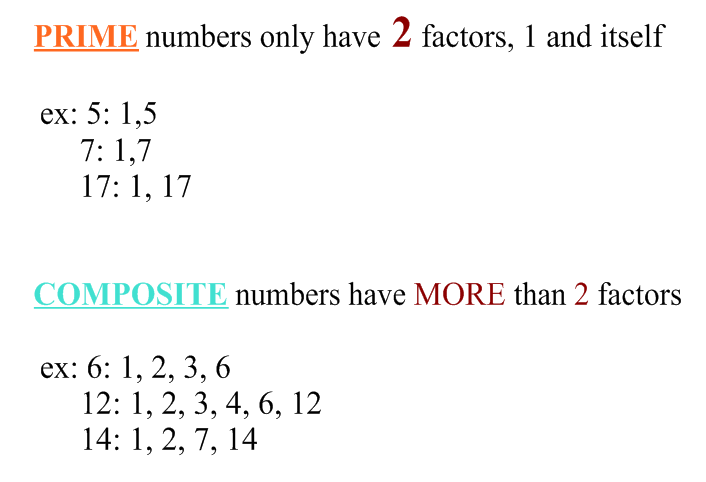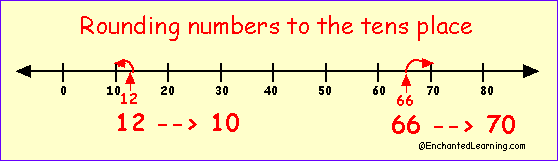# Math

### November 21- December 9:

 Standard Description Links OA 1.1 Interpret a multiplication equation as a comparison. For example, interpret 35 = 5 × 7 as a statement that 35 is 5 times as many as 7 and 7 times as many as 5. Represent verbal statements of multiplicative comparisons as multiplication equations. Videos: – Virtual Nerd: What is multiplication? – LearnZillion: Multiplicative Comparison Practice: – Multiplicative Comparison Statements – Khan Academy: Videos and Practice MD 1.1 Measurement Conversions Know relative sizes of measurement units within one system of units including km, m, cm; kg, g; lb, oz.; l, ml; hr, min, sec. Within a single system of measurement, express measurements in a larger unit in terms of a smaller unit. Record measurement equivalents in a two-column table. For example, know that 1 ft is 12 times as long as 1 in. Express the length of a 4 ft snake as 48 in. Generate a conversion table for feet and inches listing the number pairs (1, 12), (2, 24), (3, 36), … Here is a LINK to the official FSA REFERENCE SHEET that students will be allowed to use on the test. Videos: – Study Jam: Units of Measurement – Study Jam: Customary Units of Length  – Conversion Matching Games

### November 3- November 18:

 Standard Description Links NF 1.1 Equivalent Fractions Extend understanding of fraction equivalence and ordering.   Explain why a fraction a/b is equivalent to a fraction ( n x a)/(n x b) by using VISUAL FRACTION MODELS, with attention to how the number and size of the parts differ even though the two fractions themselves are the same size.  Use this to recognize/generate equivalent fractions. Videos: – Virtual Nerd: Equivalent Fractions – Brain Pop: Reducing Fractions  – Study Jams: Greatest Common Factor – Equivalent Fractions Bingo – Match Equivalent Fractions – Matching Fractions in SPACE! NF 1.2 Compare Fractions Compare two fractions with different numerators and different denominators by creating common denominators or numerators or by comparing to a benchmark fraction like ½. Recognize that comparisons are valid ONLY when the two fractions refer to the same whole. Record results w/ correct symbols & justify conclusions with a VISUAL FRACTION MODEL. Videos: – Learn Zillion: Compare FractionsPractice: – Study Jams: Compare Fractions and Mixed Numbers Games: – Order Fraction Monkeys on a Number Line

### October 20- November 2:

 Standard Description Links NF 2.3 a/b- Fractions Understanding Understand a fraction a/b with a > 1 as a sum of fractions 1/b. a. Understand addition and subtraction of fractions as joining and separating parts referring to the same whole.b. Decompose a fraction into a sum of fractions with the same denominator in more than one way, recording each decomposition by an equation. Justify decompositions, e.g., by using a visual fraction model. Examples: 3/8 = 1/8 + 1/8 + 1/8 ; 3/8 = 1/8 + 2/8 ; 2 1/8 = 1 + 1 + 1/8 = 8/8 + 8/8 + 1/8Videos: -Virtual Nerd: Add fractions -Virtual Nerd: Subtract Fractions Practice: -Study Jams: Add and Subtract fractions-Visualize Fractions Games: -Fruit Splat Addition (level 1a or 2a only)-Fraction Fling-Feed Me Fractions (addition)-FratONE (add up to 1) NF 2.3 c/d- Fractions Addition and Subtraction c. Add and subtract mixed numbers with like denominators, e.g., by replacing each mixed number with an equivalent fraction, and/or by using properties of operations and the relationship between addition and subtraction.d. Solve word problems involving addition and subtraction of fractions referring to the same whole and having like denominators, e.g., by using visual fraction models and equations to represent the problem.Videos: -Virtual Nerd: What is a mixed number? -Virtual Nerd: How do you add mixed numbers?-Virtual Nerd: How do you subtract mixed numbers?-Virtual Nerd: What’s an improper fraction?-Virtual Nerd: How do I change Mixed numbers to improper fractions? Practice: -Add Mixed Numbers (scroll down to practice) Games: -Mixed Fractions Matching

### October 3- October 19:

 Standard Description Links OA 2.4- Factors & Multiples Students will be able to Find all factor pairs for a whole number in the range 1–100. Recognize that a whole number is a multiple of each of its factors. Determine whether a given whole number in the range 1–100 is a multiple of a given one-digit number.Videos: -Virtual Nerd: What is a Factor? -Virtual Nerd: What is a Multiple? Practice: -Study Jams: Multiples -Study Jams: Divisibility Rules Games: Factors Tetris Products Partner Game OA 2.4- Prime & Composite Students will be able to determine whether a given whole number in the range 1–100 is prime or composite.Videos: -Khan Academy: Prime and Composite OA 3.5 Patterns Students will be able to generate a number or shape pattern that follows a given rule. They will identify apparent features of the pattern that were not explicit in the rule itself. Videos: -Virtual Nerd: Follow a multiplication rule -Virtual Nerd: Find a missing number in a pattern -Virtual Nerd: Use a table -Virtual Nerd: Write a rule for a patternPractice: -StudyJams: Shape Patterns -Study Jams: Number PatternsGames: – Shape Pattern Games

### September 2-September 30:

 Standard Description Links MD 1.3- Area and Perimeter Students will be able to apply the area and perimeter formulas for rectangles in real world and mathematical problems.Videos: -BrainPop: Area –BrainPop: Perimeter -Virtual Nerd: Area –Virtual Nerd: PerimeterPractice: –Khan Academy: Watch video then do two practice pages below on the side bar –Khan Academy: Watch the word problem videos then do the practice pages on the side bar. -Online GeoBoard: Make rectangles and find their area and perimeter -Interactive Activity: Area -Interactive Activity: Area and PerimeterGames: -Math Playground: Area and Perimeter -Shape Explorer: Area (click ONLY DRAW RECTANGULAR SHAPES) –Shape Explorer: Perimeter (click ONLY DRAW RECTANGULAR SHAPES) -Splash math: Area -Splash Math: Perimeter**Challenge: Party Planner NBT 2.5-Multiply Students will be able to multiply a whole number of up to four digits by a one-digit whole number using strategies based on place value and the properties of operations. They will be able to illustrate and explain using equations and area models. Videos: -Khan Academy: Area Model 4 Digit x 1 Digit –BrainPop: Multiplication –Virtual Nerd: Multiplication -Khan Academy: Different ways to multiply Practice: –StudyJam: Multiplication -Khan Academy: Watch the video then click “Multiply with Regrouping” on the side bar to practice! NBT2.6-Divide Students will be able to find whole-number quotients and remainders with up to four-digit dividends and one-digit divisors, using strategies based on place value, the properties of operations, and/or the relationship between multiplication and division. They will be able to illustrate and explain the calculation by using equations, rectangular arrays, and/or area models.Videos: -BrainPop: Division -Virtual Nerd: Division Vocabulary -LearnZillion: Divide Using Friendly Multiples -LearnZillion: Partial Quotients Practice: -StudyJam: Divisibility -StudyJam: Relating Multiplication and Division -Khan Academy: Watch the video then click “Divide Using Place Value” on the side bar to practice! –Khan Academy: Watch the Video then click “Divide by 1-Digit Numbers” on the side bar to practice! OA 1.3- Multi-Step Word Problems Students will be able to solve multi-step word problems using the four operations, including problems in which remainders must be interpreted. They will represent these problems using equations with a letter standing for the unknown.Videos: -LearnZillion: Organize data to solve problems

### August 10-September 2:

 Standard Description Links NBT 1.1- Place Value Students will be able to explain that the value of a digit gets ten times greater every time it moves one place to the left.Place Value Interactive Activity Study Jams: Place Value Virtual Nerd: Place Value NBT 1.2- Number Forms and Comparing Numbers Students will be able to read and write numbers in all three forms (standard, expanded, and word) and be able to compare and order numbers in all three forms.Virtual Nerd: Standard Form to Expanded and Word Virtual Nerd: Expanded Form to Word and Standard Virtual Nerd: Compare Two Numbers Order Numbers from Least to Greatest Order Numbers from Greatest to Least Study Jams: Order Numbers NBT 1.3- Rounding Students will be able to round whole numbers to ANY place value.Study Jams: Rounding Rounding Interactive Activity Khan Academy: Rounding Whole Numbers Place Value Quiz Game NBT 1.4- Addition and Subtraction Students will be able to fluently and accurately add and subtract multi-digit whole numbers.Virtual Nerd: Addition Virtual Nerd: Subtraction Addition and Subtraction Mission Study Jams: Addition Khan Academy: Addition Khan Academy: Subtraction LearnZillion: Addition and Subtraction Study Jams: Subtraction